POLYNOMIAL FACTOR

MAPLE can easily factor many polynomials. If you enter a polynomial with integer coefficients, then MAPLE will attempt to factor it into simpler polynomials with ineger coefficients. However, if you type 1.0 in front of one of the terms, then MAPLE will take its cue from that number and try to factor it into polynomials with rational number coefficients. You can also type in "real" or "complex" as options to tell MAPLE to use those particular types of coefficients. Additionally, MAPLE can also factor rational expressions.

> factor(x^3+5);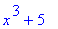> factor(1.0*x^3+5);> factor(x^3+5, real);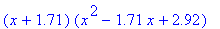> factor(x^3+5, complex);> factor((x^3+5)/(x^2-1));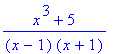> factor((1.0*x^3+5)/(x^2-1));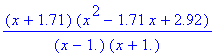> factor((x^2+2*x+1)/(x^2-1));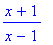> factor(x^6-x^5+x^4-x^3+x^2-x+1);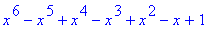> factor(x^6-x^5+x^4-x^3+x^2-x+1, real);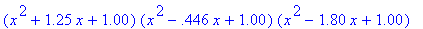> factor(x^6-x^5+x^4-x^3+x^2-x+1, complex);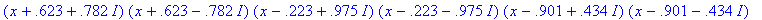> factor(x^2 - 1/4);> factor(x^2 - 1/4, real);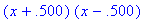>